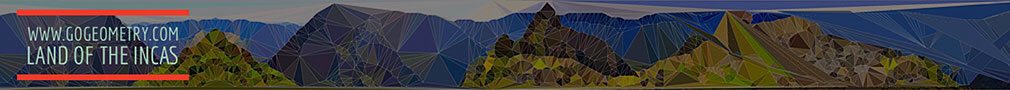# HTML5 Animation for iPad and Nexus: Nine-Point Center, Nine-Point Circle, Euler Line, Step by Step Interactive Illustration. Orthocenter, Circumcenter, Centroid, Midpoint.

Click the Next button below to start the illustration.

The Nine-point center is the center of the nine-point circle. The Nine-Point Circle of triangle ABC with orthocenter H is the circle that  passes through the feet of the altitudes HA, HB and HC to the three sides, the midpoints MA, MB and MC of those sides, and the Euler Points EA, EB and EC, which are the midpoints of the segments AH, BH, and CH, respectively. Euler line is the line passing through the orthocenter H, the nine-point center N, the centroid G, and the circumcenter O of any triangle ABC. Click the Next button above to start the step-by-step illustration.

• The nine-point center N is the midpoint of the line HO.

• The distance from the orthocenter H to the centroid G is twice the distance from the circumcenter O to the centroid G.

• The nine-point center N is the circumcenter of the medial triangle MAMBMC.

• The nine-point center N is the circumcenter of the orthic triangle HAHBHC.

The nine-point circle is also known as Euler's circle and Feuerbach's circle. Leonhard Euler showed in 1765 that the nine-point circle bisects any line from the orthocenter to a point on the circumcircle. In 1822 Karl Feuerbach discovered that any triangle's nine-point circle is externally tangent to that triangle's three excircles and internally tangent to its incircle.